Using EvolutionaryGames

Hochschule Kempten
dngebele@gmail.com
Hochschule Kempten
jochen.staudacher@hs-kempten.de

2017-11-26

Abstract

This document gives a few use cases for the EvolutionaryGames package. EvolutionaryGames provides basic concepts of evolutionary game theory, like e.g. finding evolutionary stable strategies and computing and drawing evolutionarily stable sets as well as phase diagrams for various evolutionary dynamics for single-population games with two, three and four different phenotypes.

Keywords: Evolutionary game theory, evolutionary stable strategies, evolutionarily stable sets, evolutionary game dynamics.

Motivation

The field of evolutionary game theory is concerned with interactions among large populations of strategically interacting agents. These agents may adapt their behaviour according to current payoff opportunities. Evolutionary game theory originates from biology (J. M. Smith and Price 1973), but nowadays has become an established tool in other disciplines, in particular in economics (see e.g. (Sandholm 2010)) and computer science (see e.g. (Suri 2007)).

The purpose of our package EvolutionaryGames is to enhance the R ecosystem by tools to plot and present evolutionary game dynamics in a both informative and attractive way. One similar tool in the public domain is William Sandholm’s package dynamo (Franchetti and Sandholm 2013) written in Mathematica. Dynamo (Franchetti and Sandholm 2013) frequently served as an inspiration for our implementation. Still, even though dynamo (Franchetti and Sandholm 2013) itself is free, Mathematica is proprietary software and it is nowhere near as widely used as R. Also, the package structures in R and the widespread availability of packages via CRAN make it much easier for the user to employ parts of our package EvolutionaryGames in his or her own software.

The following very brief presentation of concepts in evolutionary game theory and how EvolutionaryGames addresses them mainly follows the two books by Weibull (Weibull 1997) and Sandholm (Sandholm 2010). Furthermore, our vignette provides detailed references to the respective original articles. Our point is to show how various concepts from evolutionary game theory can be computed via the package EvolutionaryGames and where to find the conceptual, technical and mathematical details. In other words, we would like to stress that this little document is by no means intended to serve as an introduction to the fascinating field of evolutionary game theory itself. Again, for the latter the reader is referred to the the two books by Weibull (Weibull 1997) and Sandholm (Sandholm 2010). For an in-depth mathematical discussion we also recommend the book by Hofbauer and Sigmund (Hofbauer and Sigmund 1998).

The package EvolutionaryGames focusses on single-population games with two, three or four phenotypes. (The first author hopes to address multi-population games in a separate package based on EvolutionaryGames in the future.) For single-population games we regard EvolutionaryGames as a powerful and attractive alternative to William Sandholm’s Mathematica package dynamo (Franchetti and Sandholm 2013).

Computing Evolutionary Stable Strategies (ESS)

The concept of evolutionary stable strategies (ESS) was first proposed by Maynard Smith and Price in (J. M. Smith and Price 1973). An incumbent strategy $$x$$ is said to be evolutionary stable if

• it is either a unique best reply to itself or
• in case there exists an alternative best reply $$y$$ to $$x$$, then $$x$$ is a better reply to this mutant strategy $$y$$ than $$y$$ is to itself.

If $$x$$ is a strict Nash equilibrium, then $$x$$ must also be an ESS.
Using our package EvolutionaryGames one can compute ESS for both $$2$$ x $$2$$ and $$3$$ x $$3$$ symmetric matrix games. The function ESS.R receives three input arguments:

• A : A matrix specifying the symmetric game
• strategies: A vector of strings of length $$2$$ or $$3$$ specifying the names of all strategies
• floats: A logical value that handles number representation. If floats is set to TRUE (default), a floating-point number will be used for the output, otherwise the output will be specified as fractions.

Let us first take a look at a classical Hawk-Dove game.

library(EvolutionaryGames)
ESS(matrix(c(-1, 4, 0, 2), 2, byrow=TRUE), c("Hawk", "Dove"))
##           Hawk      Dove
## [1,] 0.6666667 0.3333333
ESS(matrix(c(-1, 4, 0, 2), 2, byrow=TRUE), c("Hawk", "Dove"), FALSE)
##      Hawk Dove
## [1,] 2/3  1/3

It is well known that there are games which do not possess an ESS. A classical example is the game Rock-Scissors-Paper. In such a case our function ESS.R will return NULL.

library(EvolutionaryGames)
(A <- matrix(c(1, 2, 0, 0, 1, 2, 2, 0, 1), 3, byrow=T))
##      [,1] [,2] [,3]
## [1,]    1    2    0
## [2,]    0    1    2
## [3,]    2    0    1
ESS(A, c("Rock", "Scissors", "Paper"))
## NULL

Computing and drawing evolutionarily stable sets

The concept of evolutionarily stable sets was first discussed by B. Thomas in (Thomas 1985). A very readable introduction can also be found in the book by Weibull (Weibull 1997), section 2.4.1.
An evolutionarily stable set is a nonempty closed set of symmetric Nash equilibrium strategies $$X$$ such that each strategy $$x \in X$$ earns at least the same payoff against any nearby alternative best reply $$y$$ as $$y$$ earns against itself with equal payoffs limited to the case $$y \in X$$. Note that any evolutionary stable strategy (ESS) constitutes an evolutionarily stable set and that the union of evolutionarily stable sets is again an evolutionarily stable set. See the book by Weibull (Weibull 1997), section 2.4.1, for further details.
Evolutionarily stable sets are not easy to compute and to plot. The package EvolutionaryGames computes evolutionarily stable sets of a game with two players and three strategies in the case that the game has an evolutionary stable strategy (ESS). If the two player three strategy game has no ESS, then the code returns a message stating that our algorithm cannot calculate evolutionarily stable sets for models that do not have a proper ESS. The authors are very well aware that there are games having evolutionarily stable sets but no proper ESS. Still, as our package is not devoted to finding all symmetric Nash equilibria of a game and as there currently is no package for this task on CRAN, we decided only to handle the case of games with two players and three strategies possessing at least one proper ESS. Within our algorithm, we need a proper ESS as a starting point for our computations. The authors feel that the possibility of computing and drawing evolutionarily stable sets in such cases is precious for teaching (and research) purposes. However, note that computing and drawing evolutionarily stable sets is time consuming and is clearly the most elaborate task currently performed by the package EvolutionaryGames. Finally, here is an example of an evolutionarily stable set together with a plot including sample trajectories (– the example itself is taken from the book (Broom and Rychtár 2013), p. 52):

library(EvolutionaryGames)
A <- matrix(c(-2, 5, 10/9, 0, 5/2, 10/9, -10/9, 35/9, 10/9), 3, byrow=TRUE)
strategies <- c("Hawk", "Dove", "Mixed ESS")
ESS(A, strategies)
##      Hawk Dove Mixed ESS
## [1,]    0    0         1
ESset(A, strategies)
##           [,1]      [,2] [,3]
## [1,] 0.0000000 0.0000000    1
## [2,] 0.5555556 0.4444444    0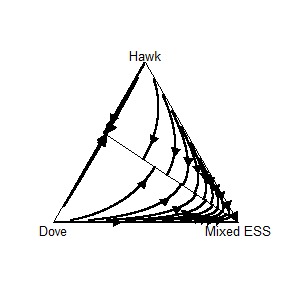The various evolutionary dynamics available in EvolutionaryGames

The basic game-theoretic model of biological natural selection is the replicator dynamic (Taylor and Jonker 1978). Still, various alternative dynamics have been proposed and investigated for different applications. Our package currently focusses on continuous dynamics only. The following itemization states which evolutionary dynamics are currently available in our package EvolutionaryGames:

• Replicator.R : The standard replicator dynamic (Taylor and Jonker 1978)
• BNN.R : The Brown-von Neumann-Nash dynamic (Brown and Neumann 1950)
• BR.R : The Best Reponse dynamic by Gilboa and Matsui (Gilboa and Matsui 1991)
• ILogit.R : The imitative Logit dynamic by Weibull (Weibull 1997). It requires the parameter eta.
• Logit.R : The Logit dynamic by Fudenberg and Levine (Fudenberg and Levine 1998). It requires the parameter eta.
• MSReplicator.R : The Maynard-Smith dynamic (J. M. Smith 1982)
• Smith.R : The Smith dynamic (M. J. Smith 1984)

Drawing phase diagrams for two, three and four strategies

Two Strategies

Drawing phase diagrams for single-population games with two, three or four phenotypes with different dynamics is the main feature of the package EvolutionaryGames. We start with a rather simple Hawk-Dove game we studied before when analyzing for its ESS. Using phaseDiagram2S.R we obtain a phase diagram for the population share of hawks invading a population of doves under the replicator dynamics.

library(EvolutionaryGames)
A <- matrix(c(-1, 4, 0, 2), 2, byrow=TRUE)
phaseDiagram2S(A, Replicator, strategies = c("Hawk", "Dove"))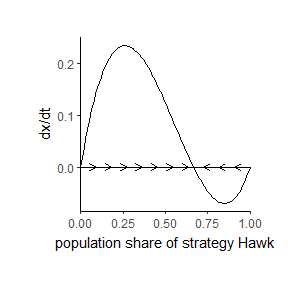For similar phase diagrams, see e.g. the book by Peters (Peters 2015).

Three Strategies

In the phase diagrams for the three strategies the user may specify the following parameters for phaseDiagram3S.R:

• A: A Numeric matrix of size $$3$$ x $$3$$ representing the number of strategies of a symmetric matrix game.
• dynamic: A function representing an evolutionary dynamic.
• params: A numeric vector with additional parameters for the evolutionary dynamic, like e.g. in the cases of the Logit or ILogit dynamics.
• trajectories: A numeric matrix of size $$m$$ x $$3$$. Each row represents the initial values for the trajectory to be examined.
• contour: A logical value that handles contour diagram presentation. By default FALSE, a nicely coloured contour plot will only be shown if the user sets contour = TRUE.
• vectorField: A logical value that handles vector field presentation. By default FALSE, a vector field will only be shown if the user sets vectorField = TRUE.
• strategies: A vector of strings of length $$3$$ specifying the names of all strategies. By default strategies = c("1","2","3").

In the following we see three plots of the game Rock-Scissors-Paper under the Replicator dynamics with the same initial state:

library(EvolutionaryGames)
A <- matrix(c(1, 2, 0, 0, 1, 2, 2, 0, 1), 3, byrow=T)
state <- matrix(c(0.7, 0.2, 0.1), 1, 3, byrow=TRUE)
RSP <- c("Rock", "Scissors", "Paper")
phaseDiagram3S(A, Replicator, NULL, state, FALSE, FALSE, strategies = RSP)
phaseDiagram3S(A, Replicator, NULL, state, TRUE, FALSE, strategies = RSP)
phaseDiagram3S(A, Replicator, NULL, state, TRUE, TRUE, strategies = RSP)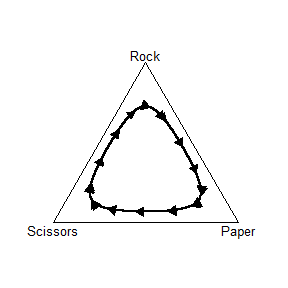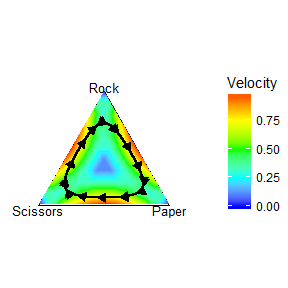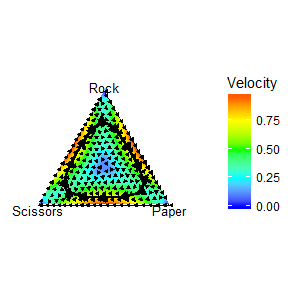Four strategies

Finally, there is also the function phaseDiagram4S.R for the case of a symmetric matrix game with four strategies. Its parameters are rather similar to those of phaseDiagram3S.R except for the facts that trajectory is a numeric vector of size $$4$$ (rather than a matrix) and that there is no possibility to enable contour plots or to plot vector fields. Instead, there is an additional logical value noRGL handling diagram rotation. By default TRUE, the diagram will only be constructed using rgl and thus become rotatable if the user specifies noRGL = FALSE. Finally, we present an example using the Smith dynamic.

library(EvolutionaryGames)
A <- matrix(c(5, -9, 6, 8, 20, 1, 2, -18, -14, 0, 2, 20, 13, 0, 4, -13), 4, 4, byrow=TRUE)
state <- c(0.6, 0.15, 0.1, 0.15)
phaseDiagram4S(A, Smith, NULL, state, noRGL=TRUE)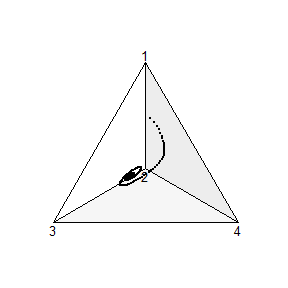How to use our package and write your own dynamics

EvolutionaryGames offers you to create your own dynamics. In particular, it is easy to write your own continuous dynamics. First of all, a dynamic is nothing other than a function that is passed as a parameter to the corresponding function for creating phase diagrams. The following code fragment shows you the minimum necessary structure of an arbitrary dynamic:

MyDynamic <- function (time, state, parameters) {

#...

return(list (dX))
}

Any dynamic requires the parameters time, state and parameters. While the parameter time is used internally by deSolve to solve the initial value problem, the other two parameters state and parameters are used to specify the desired dynamic and are available as numeric vectors. In this context, state stands for the desired initial state under which the model is to be simulated and parameters contains further parameters, such as the symmetric matrix specifying the game and, depending on the dynamic, noise levels or similar parameters.

As can be seen in the example, the return value of a specified dynamic has to be a numerical list. Each component represents the corresponding rate of change of a phenotype under the respective dynamic.

We now show how to implement a very well known dynamic, the replicator dynamics. Our function definition is as follows:

Replicator <- function (time, state, parameters) {

#...

return(list (dX))
}

The above game and an initial value is passed as a parameter to a function for the generation of phase diagrams and made retrievable within the dynamic. A small limitation, however, is that our matrix needs to be converted into a vector due to certain constraints in our internal usage of the package deSolve. We recommend that you first transfer this vector back into a matrix and maintain the original game. This can be done as follows:

Replicator <- function (time, state, parameters) {
a <- parameters
states <- sqrt(length(a))
A <- matrix(a, states, byrow = TRUE)
A <- t(A) # original symmetric game

return(list (dX))
}

We now come to the actual part of the implementation. We first calculate the rate of change of each phenotype depending on the other phenotypes. Immediately afterwards, we calculate the average fitness of each phenotype and then set up the actual replicator dynamics:

Replicator <- function(time, state, parameters) {
a <- parameters
states <- sqrt(length(a))
A <- matrix(a, states, byrow = TRUE)
A <- t(A)

dX <- c()

for(i in 1:states) {
dX[i] <- sum(state * A[i, ])
}

avgFitness <- sum(dX * state)

for(i in 1:states) {
dX[i] <- state[i] * (dX[i] - avgFitness)
}

return(list(dX))
}

Our dynamics is now applicable within the predefined functions for generating phase diagrams.

Packages used in EvolutionaryGames

We made use of various packages of the CRAN ecosystem. In particular, it was of paramount importance not to write any differential equation solvers ourselves, but to make use of an established solver, instead.

deSolve

The R-package deSolve (Soetaert, Petzoldt, and Setzer 2010) has long been established as a powerful solver for differential equations. In our context, deSolve is used to generate the data needed in order to visualize the time evolution of a given game under a certain dynamic, i.e. we use it for obtaining the correct input for the visualization of trajectories in our phase diagrams.

rgl

We use the package rgl (Adler, Murdoch, and others 2014), i.e. the R interface to OpenGL, for the four strategies case, because here the phase diagram represents a three-dimensional simplex. Our users have the possibility to follow the development of a game under a given dynamic for all phenotypes by rotating the three-dimensional simplex generated by rgl.

geometry

The R package geometry (Habel et al. 2015) helped us with the conversion from barycentric to cartesian coordinates for drawing trajectories. Solving the initial value problem returns the rates of change of the model, which have to be converted into cartesian coordinates before they can be drawn into the phase diagram in order to finally form a trajectory.

Literature

Adler, Daniel, Duncan Murdoch, and others. 2014. Rgl: 3D Visualization Device System (Opengl). https://CRAN.R-project.org/package=rgl.

Broom, Mark, and Jan Rychtár. 2013. Game-Theoretical Models in Biology. CRC Press.

Brown, G. W., and J. von Neumann. 1950. “Solutions of Games by Differential Equations.” In Contributions to the Theory of Games I, edited by Harold William Kuhn and Albert William Tucker, 73–79. Princeton University Press.

Franchetti, Francisco, and William H. Sandholm. 2013. “An Introduction to Dynamo: Diagrams for Evolutionary Game Dynamics.” Biological Theory 8 (2). Springer: 167–78.

Fudenberg, Drew, and David K. Levine. 1998. The Theory of Learning in Games. MIT Press.

Gilboa, Itzhak, and Akihiko Matsui. 1991. “Social Stability and Equilibrium”” 59: 859–67.

Habel, Kai, Raoul Grasman, Robert B. Gramacy, Andreas Stahel, and David C. Sterratt. 2015. Geometry: Mesh Generation and Surface Tesselation. https://CRAN.R-project.org/package=geometry.

Hofbauer, J., and K. Sigmund. 1998. Evolutionary Games and Population Dynamics. Cambridge University Press.

Peters, H. 2015. Game Theory: A Multi-Leveled Approach. Springer.

Sandholm, William H. 2010. Population Games and Evolutionary Dynamics. MIT Press.

Smith, John Maynard. 1982. Evolution and the Theory of Games. Cambridge University Press.

Smith, John Maynard, and George R. Price. 1973. “The Logic of Animal Conflict.” Nature 246. Springer: 15–18.

Smith, Michael J. 1984. “The Stability of a Dynamic Model of Traffic Assignment–An Application of a Method of Lyapunov” 18: 245–52.

Soetaert, Karline, Thomas Petzoldt, and R. Woodrow Setzer. 2010. “Solving Differential Equations in R: Package deSolve.” Journal of Statistical Software 33 (9): 1–25. doi:10.18637/jss.v033.i09.

Suri, S. 2007. “Computational Evolutionary Game Theory.” In Algorithmic Game Theory, edited by N. Nisan, T. Roughgarden, and E. Tardos, 717–36. Cambridge University Press.

Taylor, Peter D., and Leo B. Jonker. 1978. “Evolutionary Stable Strategies and Game Dynamics.” Mathematical Biosciences 40 (1-2). Elsevier: 145–56.

Thomas, Bernhard. 1985. “On Evolutionarily Stable Sets.” Journal of Mathematical Biology 22. Springer: 105–15.

Weibull, Jörgen W. 1997. Evolutionary Game Theory. MIT Press.